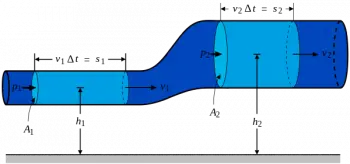# Bernoulli's Equation and Principle, Applications and ExamplesBernoulli's equation is one of the basic principles of physics and engineering that describes the relationship between the velocity of a fluid and its pressure. This principle is crucial in understanding fluid mechanics and is used in areas ranging from aerodynamics, hydrodynamics, and bridge engineering.

Bernoulli's principle is an expression of the law of energy conservation for an incompressible fluid in a steady state. Torricelli's theorem is a consequence of this principle.

## What Does Bernoulli's Principle Say?

Bernoulli's principle states that the sum of static, dynamic, and velocity pressure in an incompressible fluid is constant along a flow line.

In other words, if the velocity of a fluid increases, its pressure will decrease, and vice versa. This principle can be expressed mathematically by Bernoulli's equation, which relates a fluid's pressure, velocity, and height at a given point.

## Bernoulli's Equation

Bernoulli's equation in mathematical terms is expressed as follows:

P + 1/2*ρ*v 2 + ρ*g*h = constant

Where:

• P is the static pressure of the fluid at the point considered.

• ρ is the density of the fluid.

• v is the velocity of the fluid at the point considered.

• g is the acceleration due to gravity.

• h is the height of the considered point concerning a reference level.

The constant in the equation represents the sum of the pressures at another point in the flow line, and its value remains constant at any point along the flow line.

It is important to note that Bernoulli's equation only applies in certain situations, such as in incompressible fluids, steady state, and along a flow line. Also, the equation does not consider factors such as the fluid's viscosity or the presence of turbulence, so it may not be applicable in all situations.

## What Is the Bernoulli Equation Used For?

Bernoulli's equation is a fundamental tool in fluid mechanics and describes the behavior of fluids in various practical situations.

Some of the most essential applications of Bernoulli's equation include:

1. Piping and Conduit Design: Bernoulli's equation is used to calculate the pressure and velocity of fluid flow in pipes and conduits, which is essential in civil and mechanical engineering.

2. Wing design and aerodynamics: Bernoulli's principle is applied in the design of airplane wings and other aerodynamic devices. The shape of the wings is designed to create a pressure difference between the wings' top and bottom, allowing the planes to fly.

3. Hydraulics and Pneumatics – Along with Pascal's principle, this equation is used to design the hydraulic and pneumatic systems used in industrial machinery design.

4. Flow Measurements – This equation is also used to measure the flow of fluids in various applications, such as measuring the flow of water in a pipe or airflow in a duct.

## Examples of Bernoulli's Principle

Several experiments can be performed to demonstrate Bernoulli's principle and how velocity and pressure are related in an incompressible fluid. Some examples of Bernoulli experiments are:

### Venturi Tube

The Venturi tube is a device used to measure the velocity of a fluid. The tube consists of a conical section that tapers in the center, causing an increase in fluid velocity as it passes through the narrower section.

According to Bernoulli's principle, as the velocity of the fluid increases, the pressure decreases. The pressure difference can be measured by a manometer, which allows the fluid velocity to be calculated.

### Ping Pong Ball

If a ping-pong ball is held in a jet of air, the ball will remain suspended in the air due to the Coandă effect. This is an ideal example of how Bernoulli's principle works using the scientific method.

Air moves faster around the ball than under it, causing a drop in pressure on top of the ball, causing the ball to be pushed up.

### Airplane Wing

The design of an airplane wing is based on Bernoulli's equation. The curved shape of the wing causes air flowing over the wing to move faster than air flowing under the wing.

This creates a zone of low pressure at the top of the wing and a zone of high pressure at the bottom, generating an upward force (lift) that compensates for the aircraft's weight.

Author:

Published: April 24, 2023
Last review: April 25, 2023